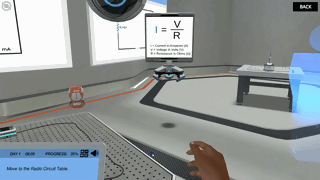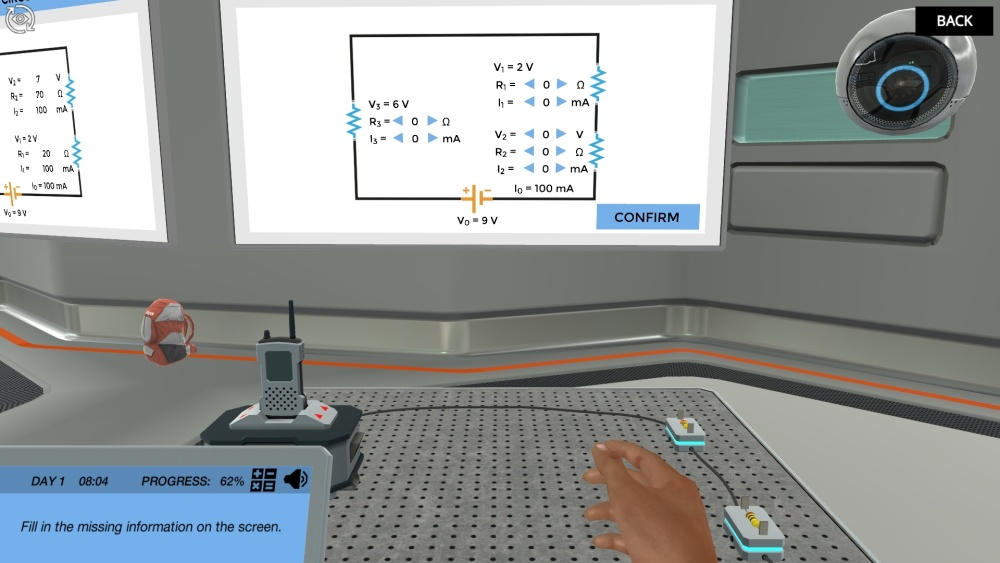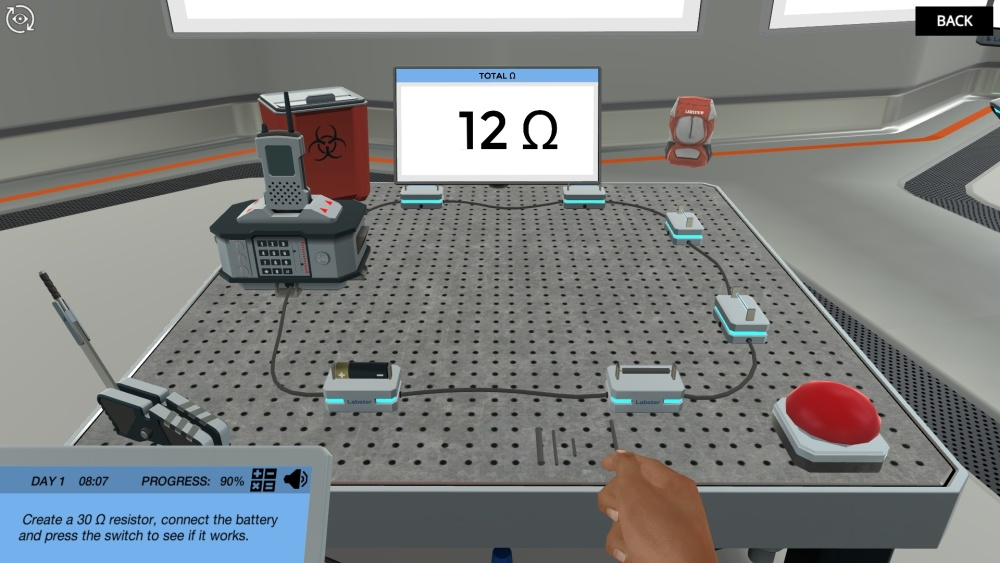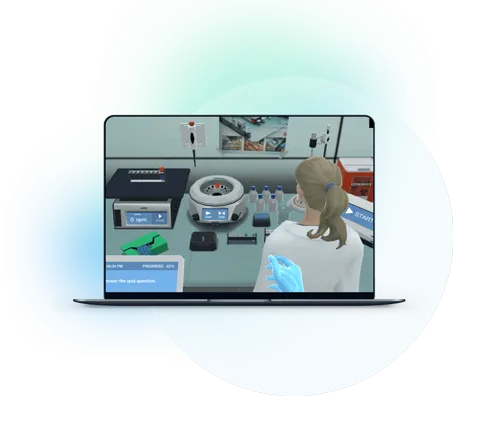Blog

# 5 Ways to Get Students Energized About Ohm’s Law

Teaching with Labster
October 11, 2022

Ohm’s is the fundamental law of electricity. It is the first law that defined the mathematical relationship between the current, voltage, and electrical resistance. Though its use case is limited to conductors only, it has set up the basis of calculation involved in designing a basic circuit. Major electrical laws in electronics are based on this law.

Laws are abstract and it is not easy to explain them to students. With multiple concepts involved, Ohm’s law is also trickier for students to understand.

Read on to learn three reasons why students find it hard, and five ways to get students energized about Ohm’s Law.GIF from Labster's Electrical Resistance and Ohm’s Law simulation.

## What Makes Ohm’s Law Trickier For Students To Understand?

Physics is an interesting subject, however, topics like electrical resistance and Ohm’s law can be tricky for students to comprehend. Here’s a list of three reasons why:

### 1. It’s Abstract

The term “Resistance” can be very abstract for students. Though students know that it means opposing some force, they fail to visualize its reference to electricity. Since it can’t be seen or felt, the students aren’t motivated to learn unless you quote some real-life applications.

### 2. It’s Complex

To understand Ohm’s law, students first have to learn the concept of resistance and resistivity. Being synonymous terms, they usually mix them up. However, as you know, resistance is the opposition to the flow of current. It is the property that depends on the material and physical condition of the conductor i.e. length, area, and temperature. Whereas, resistivity is solely a material property; it doesn’t depend on any factors.

In addition, when dealing with Ohm’s law, students come across various formulas, which can be hard for them to remember. They often mix up the calculations in series and parallel circuits due to a lack of proper knowledge and understanding.

### 3. Circuit Diagrams are hard to solve

You are teaching Ohm’s law and there is no way, you won’t discuss the circuit diagrams. The circuit diagrams are the actual application of Ohm’s law. However, for students, finding the unknown values of current, voltage and resistance can be hard.

Moreover, mostly when parallel and series circuits get combined, it becomes even harder for them to sort out things and solve these circuits.Snippet from Labster’s Electrical Resistance and Ohm’s Law simulation.

## 5 Ways To Make Ohm’s Law A More Approachable Topic

Keeping in view some of the things that students might cause trouble for students, here are five ways through which you can make the topic more fun for students.

### 1. Show Them People Who Discovered it

Students might not show any interest in learning this theoretical law until you tell them how to narrate the law in the form of a story. For instance, you can tell them about the scientist who discovered it and how he came to that conclusion.

Stories often create interest in the subject, and students usually associate the characters with the topic in question. This helps them remember the topic for the long term.  In the current case, you can mention the life story of George Simon Ohm who proposed Ohm’s law.

The Man Behind Ohm’s Law: George Simon Ohm

George Simon Ohm was a German mathematics teacher, who used to teach in local schools. Besides that, he also experimented in the school's Physics laboratory. His career took a leap when he was appointed a professor of Mathematics at Jesuit College in 1817.

Since he was very good at mathematics, he thought of developing a mathematical model for an electrical circuit. But at that time, there weren’t any devices to measure current or voltage. The only resources available were the Oersted theory of electromagnetism to detect current, the electrochemical cell of Volta, and Seebeck’s thermocouple.

With all available resources, he designed a very interesting experimental setup. He used two tiny vessels; one with boiling water at 100 ℃, and the other with snow at 0 ℃. The idea was to create a thermocouple, which he accomplished using two copper and one bismuth strip.

For electric current to flow through the circuit, he placed one end of copper into boiling water and the other end into the snow, as shown in the schematic diagram. Since he need to test the current on multiple conductors, he inserted a test conductor (which could be altered) into two cups of mercury.

As the current flowed through the circuit, the compass needle, consisting of metal wire, deflected due to the magnetic field. A torsion element was used to restore the needle back to its position. The amount of current was calculated in terms of torsion required to restore the needle to its resting position.

To vary the resistance, Ohm used test conductors of different lengths and materials. For the findings of his experiment, he deduced that current passing through a conductor is directly proportional to the voltage applied across its ends and inversely proportional to the resistance of the conductor.

This law was published in his book, Die Galvanische Kette mathematisch beavbeitet, but wasn’t perceived very well among scientists.  One professor from Berlin, Prof. Dave, called it a naked web of fancies. However, with time, when the researchers realized the worth of this law. He was awarded the Copley Medal in 1841. Later after his death, the unit of electrical resistance, Ohm, was named after him.

### 2. Use Physical Analogies to Explain Abstract Concepts

To make abstract concepts simpler, you can use different analogies. To explain Ohm’s law, the best analogy can be water circulating in pipes.  Consider that you have a pump that pushes water down a pipe which has a restriction whose size can be varied. In this case, the pump’s pressure is voltage, the flow rate of water is current, the restriction is the pipe is resistance, and the valve acts as the circuit switch.

Image Source: Xaktly

Now, keeping the resistance to flow constant, if we increase the pump’s pressure (voltage), the flow rate will increase (current). That’s the statement of Ohm’s law: current in the conducting wire is directly proportional to voltage.

↑V = ↑I  .  R (const.)

Similarly, if we keep the pressure (voltage) constant, but increase the size of the restriction i.e. make it too difficult to flow, the flow rate will obviously decrease.

V const.= I  .  ↑ R

Lastly, if the flow rate (current) is to be kept constant, a small restriction (resistance) would require less pressure from the pump (voltage).

↓V =  I (const.).  ↓R

### 3. Take Help from Ohm’s Pyramid

Some students have trouble memorizing the formula even if they are quite simple. For Ohm’s law, as you know, three forms exist.

V=IR, I= V/R, R=V/I

An easy way to remember these three formulas is by drawing an Ohm’s pyramid or Ohm’s triangle. First, make a triangle and divide it into three sections. On the top corner of the pyramid, write V. On the bottom left corner, write I, and right-left corner, write R.

Now, use the triangle to find the unknown. Circle the unknown, and drive its formula from the position of the other two.  If the two values are inline, they are multiplied. However, if they are one on top of another, they are divided. For instance, I is equal to V over R since V is on the top of R. With this pyramid, students can easily figure out how to find the unknowns of the circuits.

### 4. Mention their Real-World Applications

Ohm’s law and resistance concepts are abstract and boring. Students usually ask out their teachers where we apply this law. Where do we use these formulas? Mentioning some real-world applications, that students can relate to, excites them to learn about them.

Show them Resistors

Most students won’t have seen resistors, they just know them from a circuit diagram in their textbooks. You can show them tiny resistors used in the remote of an appliance. You can tell them why resistors are an integral part of the circuits.

Let Them Solve Scenario based Problems

Moreover, with some numerical problems, you can show them how Ohm’s law is used to design all electric circuits. For instance, you can assign them a simple problem to solve:

“What will be the resistance of the wire, if a current of 2 A passes through it when a potential difference of 100 Volts is applied across its ends.

Explain the Type of Circuit Used in Homes

You can explain to students why all of our home appliances are connected in parallel, not in series. Because we want every appliance to run on the same voltage (220-240 V in homes) and independently turn ON and OFF.

If the devices were connected in series, firstly, the voltage would have been divided, and secondly, if one of the appliances failed, the entire circuit would have broken. Moreover, in a series circuit, the device cannot be switched ON and OFF.

### 5. Use Virtual Lab Simulations

Visualizing the process is the best way to understand it. That’s where the virtual lab simulations can work. You can show the students interactive animations to make things more interesting.Check out Labster’s Electrical Resistance and Ohm’s Law simulation. In this simulation, students learn how changing resistance affects the current. They get to build their circuit to repair a broken radio.

At Labster, we engage students in science by having them go through different 3D scenarios. The web-based simulation can be played on any device, enhancing long-term learning outcomes. If you are interested in Labster simulations for your students or want to explore more, contact us now.What exactly are virtual labs?